The Central Limit Theorem

# 34 The Central Limit Theorem for Sample Means

The sampling distribution is a theoretical distribution. It is created by taking many many samples of size n from a population. Each sample mean is then treated like a single observation of this new distribution, the sampling distribution. The genius of thinking this way is that it recognizes that when we sample we are creating an observation and that observation must come from some particular distribution. The Central Limit Theorem answers the question: from what distribution did a sample mean come? If this is discovered, then we can treat a sample mean just like any other observation and calculate probabilities about what values it might take on. We have effectively moved from the world of statistics where we know only what we have from the sample, to the world of probability where we know the distribution from which the sample mean came and the parameters of that distribution.

The reasons that one samples a population are obvious. The time and expense of checking every invoice to determine its validity or every shipment to see if it contains all the items may well exceed the cost of errors in billing or shipping. For some products, sampling would require destroying them, called destructive sampling. One such example is measuring the ability of a metal to withstand saltwater corrosion for parts on ocean going vessels.

Sampling thus raises an important question; just which sample was drawn. Even if the sample were randomly drawn, there are theoretically an almost infinite number of samples. With just 100 items, there are more than 75 million unique samples of size five that can be drawn. If six are in the sample, the number of possible samples increases to just more than one billion. Of the 75 million possible samples, then, which one did you get? If there is variation in the items to be sampled, there will be variation in the samples. One could draw an “unlucky” sample and make very wrong conclusions concerning the population. This recognition that any sample we draw is really only one from a distribution of samples provides us with what is probably the single most important theorem is statistics: the Central Limit Theorem. Without the Central Limit Theorem it would be impossible to proceed to inferential statistics from simple probability theory. In its most basic form, the Central Limit Theorem states that regardless of the underlying probability density function of the population data, the theoretical distribution of the means of samples from the population will be normally distributed. In essence, this says that the mean of a sample should be treated like an observation drawn from a normal distribution. The Central Limit Theorem only holds if the sample size is “large enough” which has been shown to be only 30 observations or more.

(Figure) graphically displays this very important proposition.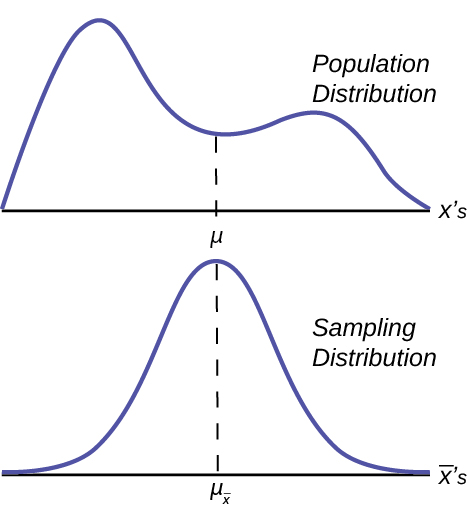Notice that the horizontal axis in the top panel is labeled X. These are the individual observations of the population. This is the unknown distribution of the population values. The graph is purposefully drawn all squiggly to show that it does not matter just how odd ball it really is. Remember, we will never know what this distribution looks like, or its mean or standard deviation for that matter.

The horizontal axis in the bottom panel is labeled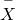‘s. This is the theoretical distribution called the sampling distribution of the means. Each observation on this distribution is a sample mean. All these sample means were calculated from individual samples with the same sample size. The theoretical sampling distribution contains all of the sample mean values from all the possible samples that could have been taken from the population. Of course, no one would ever actually take all of these samples, but if they did this is how they would look. And the Central Limit Theorem says that they will be normally distributed.

The Central Limit Theorem goes even further and tells us the mean and standard deviation of this theoretical distribution.

Parameter Population distribution Sample Sampling distribution of‘s
Mean μ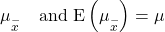Standard deviation σ s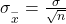The practical significance of The Central Limit Theorem is that now we can compute probabilities for drawing a sample mean,, in just the same way as we did for drawing specific observations, X’s, when we knew the population mean and standard deviation and that the population data were normally distributed.. The standardizing formula has to be amended to recognize that the mean and standard deviation of the sampling distribution, sometimes, called the standard error of the mean, are different from those of the population distribution, but otherwise nothing has changed. The new standardizing formula is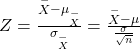Notice that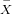in the first formula has been changed to simply µ in the second version. The reason is that mathematically it can be shown that the expected value ofis equal to µ. This was stated in (Figure) above. Mathematically, the E(x) symbol read the “expected value of x”. This formula will be used in the next unit to provide estimates of the unknown population parameter μ.

### References

Baran, Daya. “20 Percent of Americans Have Never Used Email.”WebGuild, 2010. Available online at http://www.webguild.org/20080519/20-percent-of-americans-have-never-used-email (accessed May 17, 2013).

Data from The Flurry Blog, 2013. Available online at http://blog.flurry.com (accessed May 17, 2013).

Data from the United States Department of Agriculture.

### Chapter Review

In a population whose distribution may be known or unknown, if the size (n) of samples is sufficiently large, the distribution of the sample means will be approximately normal. The mean of the sample means will equal the population mean. The standard deviation of the distribution of the sample means, called the standard error of the mean, is equal to the population standard deviation divided by the square root of the sample size (n).

### Formula Review

The Central Limit Theorem for Sample Means:~ N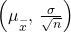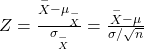The Mean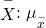Central Limit Theorem for Sample Means z-score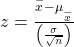Standard Error of the Mean (Standard Deviation ()):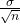Finite Population Correction Factor for the sampling distribution of means: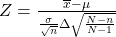Finite Population Correction Factor for the sampling distribution of proportions: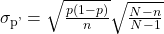### Homework

Previously, De Anza statistics students estimated that the amount of change daytime statistics students carry is exponentially distributed with a mean of ?0.88. Suppose that we randomly pick 25 daytime statistics students.

1. In words, Χ = ____________
2. Χ ~ _____(_____,_____)
3. In words,= ____________
4.~ ______ (______, ______)
5. Find the probability that an individual had between ?0.80 and ?1.00. Graph the situation, and shade in the area to be determined.
6. Find the probability that the average of the 25 students was between ?0.80 and ?1.00. Graph the situation, and shade in the area to be determined.
7. Explain why there is a difference in part e and part f.
1. Χ = amount of change students carry
2. Χ ~ E(0.88, 0.88)
3.= average amount of change carried by a sample of 25 students.
4.~ N(0.88, 0.176)
5. 0.0819
6. 0.1882
7. The distributions are different. Part a is exponential and part b is normal.

Suppose that the distance of fly balls hit to the outfield (in baseball) is normally distributed with a mean of 250 feet and a standard deviation of 50 feet. We randomly sample 49 fly balls.

1. If= average distance in feet for 49 fly balls, then~ _______(_______,_______)
2. What is the probability that the 49 balls traveled an average of less than 240 feet? Sketch the graph. Scale the horizontal axis for. Shade the region corresponding to the probability. Find the probability.
3. Find the 80th percentile of the distribution of the average of 49 fly balls.

<!– <solution id=”id6274098″> N ( 250,  50 49 ) 0.0808 256.01 feet –>

According to the Internal Revenue Service, the average length of time for an individual to complete (keep records for, learn, prepare, copy, assemble, and send) IRS Form 1040 is 10.53 hours (without any attached schedules). The distribution is unknown. Let us assume that the standard deviation is two hours. Suppose we randomly sample 36 taxpayers.

1. In words, Χ = _____________
2. In words,= _____________
3.~ _____(_____,_____)
4. Would you be surprised if the 36 taxpayers finished their Form 1040s in an average of more than 12 hours? Explain why or why not in complete sentences.
5. Would you be surprised if one taxpayer finished his or her Form 1040 in more than 12 hours? In a complete sentence, explain why.
1. length of time for an individual to complete IRS form 1040, in hours.
2. mean length of time for a sample of 36 taxpayers to complete IRS form 1040, in hours.
3. N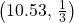4. Yes. I would be surprised, because the probability is almost 0.
5. No. I would not be totally surprised because the probability is 0.2312

Suppose that a category of world-class runners are known to run a marathon (26 miles) in an average of 145 minutes with a standard deviation of 14 minutes. Consider 49 of the races. Letthe average of the 49 races.

1.~ _____(_____,_____)
2. Find the probability that the runner will average between 142 and 146 minutes in these 49 marathons.
3. Find the 80th percentile for the average of these 49 marathons.
4. Find the median of the average running times.

<!– <solution id=”id6533816″> N ( 145,  14 49 ) 0.6247 146.68 145 minutes –>

The length of songs in a collector’s iTunes album collection is uniformly distributed from two to 3.5 minutes. Suppose we randomly pick five albums from the collection. There are a total of 43 songs on the five albums.

1. In words, Χ = _________
2. Χ ~ _____________
3. In words,= _____________
4.~ _____(_____,_____)
5. Find the first quartile for the average song length.
6. The IQR(interquartile range) for the average song length is from _______–_______.
1. the length of a song, in minutes, in the collection
2. U(2, 3.5)
3. the average length, in minutes, of the songs from a sample of five albums from the collection
4. N(2.75, 0.066)
5. 2.74 minutes
6. 0.03 minutes

In 1940 the average size of a U.S. farm was 174 acres. Let’s say that the standard deviation was 55 acres. Suppose we randomly survey 38 farmers from 1940.

1. In words, Χ = _____________
2. In words,= _____________
3.~ _____(_____,_____)
4. The IQR foris from _______ acres to _______ acres.

<!– <solution id=”fs-idm55467904″> the size of a U.S. farm in 1940 the average size of a U.S. farm, in acres N ( 174,  55 38 ) 168.0, 180.0 –>

Determine which of the following are true and which are false. Then, in complete sentences, justify your answers.

1. When the sample size is large, the mean ofis approximately equal to the mean of Χ.
2. When the sample size is large,is approximately normally distributed.
3. When the sample size is large, the standard deviation ofis approximately the same as the standard deviation of Χ.
1. True. The mean of a sampling distribution of the means is approximately the mean of the data distribution.
2. True. According to the Central Limit Theorem, the larger the sample, the closer the sampling distribution of the means becomes normal.
3. The standard deviation of the sampling distribution of the means will decrease making it approximately the same as the standard deviation of X as the sample size increases.

The percent of fat calories that a person in America consumes each day is normally distributed with a mean of about 36 and a standard deviation of about ten. Suppose that 16 individuals are randomly chosen. Let= average percent of fat calories.

1.~ ______(______, ______)
2. For the group of 16, find the probability that the average percent of fat calories consumed is more than five. Graph the situation and shade in the area to be determined.
3. Find the first quartile for the average percent of fat calories.

<!– <solution id=”id6272525″> N ( 36,  10 16 ) 1 34.31 –>

The distribution of income in some Third World countries is considered wedge shaped (many very poor people, very few middle income people, and even fewer wealthy people). Suppose we pick a country with a wedge shaped distribution. Let the average salary be ?2,000 per year with a standard deviation of ?8,000. We randomly survey 1,000 residents of that country.

1. In words, Χ = _____________
2. In words,= _____________
3.~ _____(_____,_____)
4. How is it possible for the standard deviation to be greater than the average?
5. Why is it more likely that the average of the 1,000 residents will be from ?2,000 to ?2,100 than from ?2,100 to ?2,200?
1. X = the yearly income of someone in a third world country
2. the average salary from samples of 1,000 residents of a third world country
3.N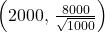4. Very wide differences in data values can have averages smaller than standard deviations.
5. The distribution of the sample mean will have higher probabilities closer to the population mean.
P(2000 << 2100) = 0.1537
P(2100 << 2200) = 0.1317

Which of the following is NOT TRUE about the distribution for averages?

1. The mean, median, and mode are equal.
2. The area under the curve is one.
3. The curve never touches the x-axis.
4. The curve is skewed to the right.

<!– <solution id=”id6538477″> d –>

The cost of unleaded gasoline in the Bay Area once followed an unknown distribution with a mean of ?4.59 and a standard deviation of ?0.10. Sixteen gas stations from the Bay Area are randomly chosen. We are interested in the average cost of gasoline for the 16 gas stations. The distribution to use for the average cost of gasoline for the 16 gas stations is:

1.~ N(4.59, 0.10)
2.~ N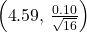3.~ N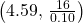4.~ N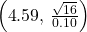b

### Key Terms

Average
a number that describes the central tendency of the data; there are a number of specialized averages, including the arithmetic mean, weighted mean, median, mode, and geometric mean.
Central Limit Theorem
Given a random variable with known mean μ and known standard deviation, σ, we are sampling with size n, and we are interested in two new RVs: the sample mean,. If the size (n) of the sample is sufficiently large, then~ N(μ,). If the size (n) of the sample is sufficiently large, then the distribution of the sample means will approximate a normal distributions regardless of the shape of the population. The mean of the sample means will equal the population mean. The standard deviation of the distribution of the sample means,, is called the standard error of the mean.
Standard Error of the Mean
the standard deviation of the distribution of the sample means, or.